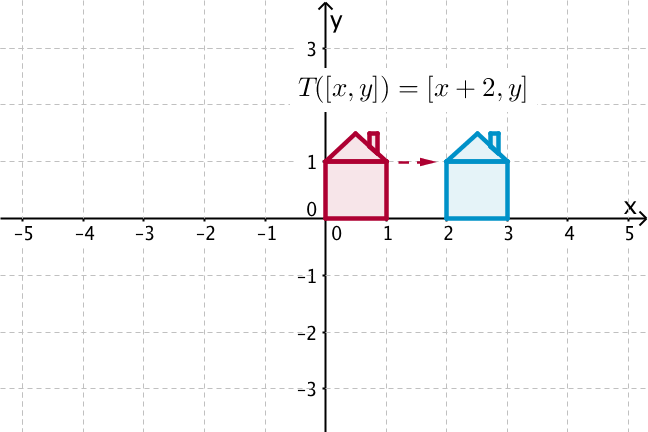## Want to keep learning?

This content is taken from the UNSW Sydney's online course, Maths for Humans: Linear, Quadratic & Inverse Relations. Join the course to learn more.
1.11

## UNSW Sydney# Translations, reflections and dilations

The explicit and concrete aspect of the Cartesian plane allows us to be precise when talking about planar transformations. Here we introduce translations, reflections and dilations.

In this step you will get a good visual and algebraic understanding of several types of transformations in the Cartesian plane.

## Translations

Becoming familiar with how to represent transformations is vital for computer artists, architects, engineers and video game designers. To see what a transformation does, it is convenient to think about the image of a little house, with corners $$\normalsize{[0, 0]}$$, $$\normalsize{[1, 0]}$$, $$\normalsize{[1, 1]}$$ and $$\normalsize{[0, 1]}$$, and apex of the roof at $$\normalsize{[1/2,3/2]}$$. Note that the house has a chimney too (which will allow us to know when the image of the house has been flipped).

Let’s see what happens to our little “unit house” if we apply some simple transformations.The map $$\normalsize{T:[x,y]}$$ to $$\normalsize{[x+2,y]}$$ is a translation by two in the (positive) $$\normalsize{x}$$ direction. Every point gets moved by exactly the same amount.The map $$\normalsize{U:[x,y]}$$ to $$\normalsize{[x,y-3]}$$ is a translation by negative three in the $$\normalsize y$$ direction. Every points gets moved by exactly the same amount.The map $$\normalsize{V:[x,y]}$$ to $$\normalsize{[x+2,y-3]}$$ is a combination of a translation by $$\normalsize{2}$$ in the $$\normalsize{x}$$ direction, and a translation by $$\normalsize{-3}$$ in the $$\normalsize{y}$$ direction.## Reflections

The transformation $$\normalsize{R:[x,y]}$$ to $$\normalsize{[x,-y]}$$ sends every point to its mirror image in the $$\normalsize{x}$$ axis. If we perform this transformation twice, we get back to where we started.The transformation $$\normalsize{S: [x,y]}$$ to $$\normalsize{[-x,y]}$$ sends every point to its reflection in the $$\normalsize{y}$$ axis.We can also, with a bit of experience, get at more complicated reflections by specifying what they do to points. The aim is to find the axis of reflection — that is, the mirror in which points are being reflected.

Q1 (E): Consider the transformation $$\normalsize{E: [x,y]}$$ to $$[2-x,y]$$. What does it do? We can get at this by having a look at what happens to some specific points. What happens to $$\normalsize{[0,0]}$$? to $$\normalsize{[1,0]}$$? to $$\normalsize{[0,1]}$$? to $$\normalsize{[2,3]}$$? If we graph these, perhaps we can get an inkling for what is going on.

Q2 (M): Another rather important reflection is this one: $$\normalsize{F:[x,y]}$$ to $$\normalsize{[y,x]}$$. By graphing some points, try to figure out what is happening here geometrically.

Q3 (C): What is the equation for a reflection $$\normalsize{G}$$ in the line $$\normalsize{y=3}$$?

Q4 (M): What is the equation for a reflection $$\normalsize{H}$$ in the line $$\normalsize{y=-x}$$?

## Dilations

A dilation is a stretch by some constant fixed factor in some direction. The factor can be bigger than one, in which case it expands, or it could be by a positive factor less than one, in which case we have a shrink.

For example $$\normalsize{D: [x,y]}$$ to $$\normalsize{[3x,y]}$$ is a dilation by $$\normalsize{3}$$ in the $$\normalsize{x}$$ direction, while $$\normalsize{E: [x,y]}$$ to $$\normalsize{[x,y/2]}$$ is a dilation by $$\normalsize{1/2}$$ in the $$\normalsize{y}$$ direction. We can stretch (with $$\normalsize D$$) and then squash (with $$\normalsize E$$) the house by combining these dilations.A true dilation affects both coordinates equally.Q5 (M): Describe what would happen to our unit house after applying a transformation $$\normalsize{J}: [x,y]$$ to $$[x/2, 2y + 3]$$.

A1. The transformation is the reflection in the vertical line with equation $$\normalsize{x=1}$$.

A2. This is reflection in the line $$\normalsize{y=x}$$, which passes through the origin $$\normalsize{[0,0]}$$ and the point $$\normalsize{[1,1]}$$.

A3. It is perhaps easier to start in one dimension. Suppose we had a line with coordinate $$\normalsize{y}$$, and we wanted to consider the reflection say $$\normalsize{r}$$ about the point $$\normalsize{3}$$. Then for example $$\normalsize{r(3)=3}$$, $$\normalsize{r(2)=4}$$, $$\normalsize{r(1)=5}$$ and $$\normalsize{r(8)=-2}$$.

What is the general formula? Note that the point $$\normalsize{y}$$ and its image $$\normalsize{r(y)}$$ are equally spaced around $$\normalsize{3}$$, so their midpoint is exactly $$\normalsize{y=3}$$. That means that

$\Large{\frac{y+r(y)}{2}=3}.$

But from that it follows that

$\Large{r(y)=6-y}.$

So going back to the reflection in the line $$\normalsize{y=3}$$; that is going to be the transformation that sends $$\normalsize{[x,y]}$$ to $$\normalsize{[x,6-y]}$$.

A4. This is the transformation $$\normalsize{H: [x,y]}$$ to $$\normalsize{[-y,-x]}$$.

A5. $$\normalsize{J}: [x,y]$$ to $$[x/2, 2y + 3]$$ results in a dilation by a factor of 1/2 in the $$\normalsize x$$ direction, a dilation by a factor of 2 in the $$\normalsize y$$ direction, and a translation by 3 in the $$\normalsize y$$ direction.